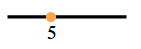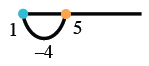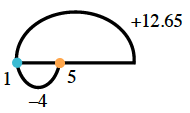### Home > CC1 > Chapter Ch4 > Lesson 4.1.1 > Problem4-9

4-9.

Simplify each expression below. For each expression, draw a picture or show how you know your answer makes sense.

1. $5+(-4)+12.65$

• Use a drawing such as the one to the right to help you make sense of the problem. A number line with a point, labeled, 5.

• Starting at $5$, add $-4$. In other words, subtract 4. Added to the number line, an arc connecting 1 to 5, with a label of negative 4.

• Now add $12.65$Added to previous number line, an arc from 1 extending to the right, with label, plus 12.65.

• $13.65$1. $6.5+(-2)+10.5$

• See part (a).

1. $4(-5+100)$

Add what is in the parentheses first, then multiply by 4.

2. $-212+(-102)$

Use a drawing as in part (a) to help make sense of this problem.

3. $4+6(3)+2(5\frac{1}{2}-1)$

Remember to subtract what is in the parentheses first before multiplying by 2.

Make sure all multiplication is done before adding.

$31$

4. $5+3(5)+(-4)(5)$